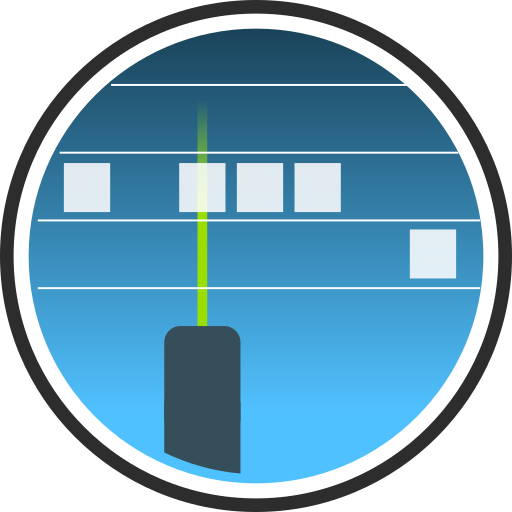#ALCF

## Command line interface

After completing the installation, the ALCF commands can be run in the terminal with the `alcf` program. The program uses the command line argument format PST for passing complex command line arguments.

### Commands

Command Description
alcf the main command
alcf auto peform automatic processing of model or lidar data
alcf calibrate calibrate lidar backscatter
alcf convert convert input instrument or model data to the ALCF standard NetCDF
alcf lidar process lidar data
alcf model extract model data at a point or along a track
alcf plot plot lidar data
alcf simulate simulate lidar measurements from model data using COSP
alcf stats calculate cloud occurrence statistics

### Automatic processing

Automatic processing of lidar measurements can be done with the `alcf auto lidar` command and performs resampling, noise removal, cloud detection, calculates cloud occurrence and backscatter histograms and plots backscatter profiles, cloud occurrence and the backscatter histogram. `alcf auto lidar` does not perform initial data conversion from raw instrument data to NetCDF, and this needs to be done manually by running `alcf convert` for instruments which do not produce NetCDF output.

Automatic processing of model data can be done with the `alcf auto model` command and performs extraction of model data, runs the lidar simulator, and processes the simulated backscatter in the same way as `alcf lidar`.

### Manual processing

The commands are usually run in the following order.

ALC observations processing:

1. `alcf convert` – convert raw ALC data to NetCDF (only if not in NetCDF already),
2. `alcf lidar` – produce resampled data,
3. `alcf plot backscatter` – plot backscatter profiles,
4. `alcf stats` – calculate summary statistics from resampled lidar data from step 2.,
5. `alcf plot cloud_occurrence` – plot cloud occurrence calculated in step 4.,
6. `alcf plot backscatter_hist` – plot backscatter histogram calculated in step 4.

Model output processing:

1. `alcf model` – extract model data at a geographical point or along a ship track,
2. `alcf simulate` – simulate backscatter based on data from step 1.,
3. `alcf lidar` – resample simulated backscatter data from step 2.,
4. `alcf plot backscatter` – plot simulated backscatter profiles from step 3.,
5. `alcf stats` – calculate summary statistics from resampled simulated backscatter data from step 3.,
6. `alcf plot cloud_occurrence` – plot cloud occurrence calculated in step 5.,
7. `alcf plot backscatter_hist` – plot backscatter histogram calculated in step 5.

NetCDF data files generated in each step are described in the ALCF output and can be previewed in Panoply.